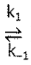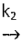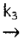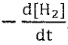# Problem: The proposed mechanism for a given reaction is as follows:Step One: 2 NO (g)    N2O2 (g)Step Two: H2(g) + N2O2(g)    H2O(g) + N2O(g)      Rate - determining StepStep Three: N2O(g) + H2(g)    N2(g) + H2O(g)    FastUse the steady-state approximation on intermediates to write the rate law for this reaction in terms of  . Your law should include only elementary rate constants and species that appear in the overall reaction. Show all work to support your reasoning and answer. Note: you may Not assume step one is a fast pre-equilibrium.

###### FREE Expert Solution

For this problem, we have to write the rate law for this reaction in terms of -d[H2]/dt using steady-state approximation

Recall that the rate law only focuses on the reactant concentrations and has a general form of:

k = rate constant
A & B = reactants
x & y = reactant orders

• A steady-state approach makes use of the assumption that the rate of production of an intermediate is equal to the rate of its consumption.
• We will be starting this with:

96% (466 ratings)###### Problem Details

The proposed mechanism for a given reaction is as follows:

Step One: 2 NO (g)N2O2 (g)

Step Two: H2(g) + N2O2(g)H2O(g) + N2O(g)      Rate - determining Step

Step Three: N2O(g) + H2(g)N2(g) + H2O(g)    Fast

Use the steady-state approximation on intermediates to write the rate law for this reaction in terms of. Your law should include only elementary rate constants and species that appear in the overall reaction. Show all work to support your reasoning and answer. Note: you may Not assume step one is a fast pre-equilibrium.